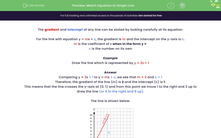# Find Equations for Straight Line Graphs

In this worksheet, students will find the correct equation for a straight line graph.Key stage:  KS 3

Curriculum topic:   Algebra

Curriculum subtopic:   Translate Situations/Procedures into Expressions, Formulae and Graphs

Popular topics:   Algebra worksheets

Difficulty level:#### Worksheet Overview

The gradient and intercept of any line can be stated by looking carefully at its equation.

For the line with equation y = mx + c, the gradient is m and the intercept on the y-axis is c.

m is the coefficient of x when in the form y =

c is the number on its own

Example

Draw the line which is represented by y = 2x + 1

Comparing y = 2x + 1 to y = mx + c, we see that m = 2 and c = 1

Therefore, the gradient of the line (m) is 2 and the intercept (c) is 1.

This means that the line crosses the y-axis at (0, 1)

The gradient of a line means the vertical ÷ horizontal. So, with a gradient of 2, we must have a line that goes 2 up and 1 to the right of the y intercept (0,1)

This gives us a point of (1,3). If we draw a straight line that passes through (0,1) and (1,3) we should have drawn the line for the equation y = 2x + 1

The line is shown below:Let's tackle some questions together.

Remember, you can always refer back to this page for guidance if you need to - just click on the red button.

### What is EdPlace?

We're your National Curriculum aligned online education content provider helping each child succeed in English, maths and science from year 1 to GCSE. With an EdPlace account you’ll be able to track and measure progress, helping each child achieve their best. We build confidence and attainment by personalising each child’s learning at a level that suits them.

Get started